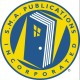## National new home metrics for July; 2021

Building Permits

Privately‐owned housing units authorized by building permits in July were at a seasonally adjusted annual rate of 1,635,000. This is 2.6 percent (±0.9 percent) above the revised June rate of 1,594,000 and is 6.0 percent (±0.9 percent) above the July 2020 rate of 1,542,000. Single‐family authorizations in July were at a rate of 1,048,000; this is 1.7 percent (±0.8 percent) below the revised June figure of 1,066,000.

Authorizations of units in buildings with five units or more were at a rate of 532,000 in July.

Housing Starts

Privately‐owned housing starts in July were at a seasonally adjusted annual rate of 1,534,000. This is 7.0 percent (±8.9 percent)* below the revised June estimate of 1,650,000, but is 2.5 percent (±10.9 percent)* above the July 2020 rate of 1,497,000. Single‐family housing starts in July were at a rate of 1,111,000; this is 4.5 percent (±9.9percent)* below the revised June figure of 1,163,000. The July rate for units in buildings with five units or more was 412,000.

Housing Completions

Privately‐owned housing completions in July were at a seasonally adjusted annual rate of 1,391,000. This is 5.6 percent (±16.4 percent)* above the revised June estimate of 1,317,000 and is 3.8 percent (±14.4 percent)* above the July 2020 rate of 1,340,000. Single‐family housing completions in July were at a rate of 954,000; this is 3.6 percent (±16.1 percent)* above the revised June rate of 921,000. The July rate for units in buildings with five units or more was 426,000.